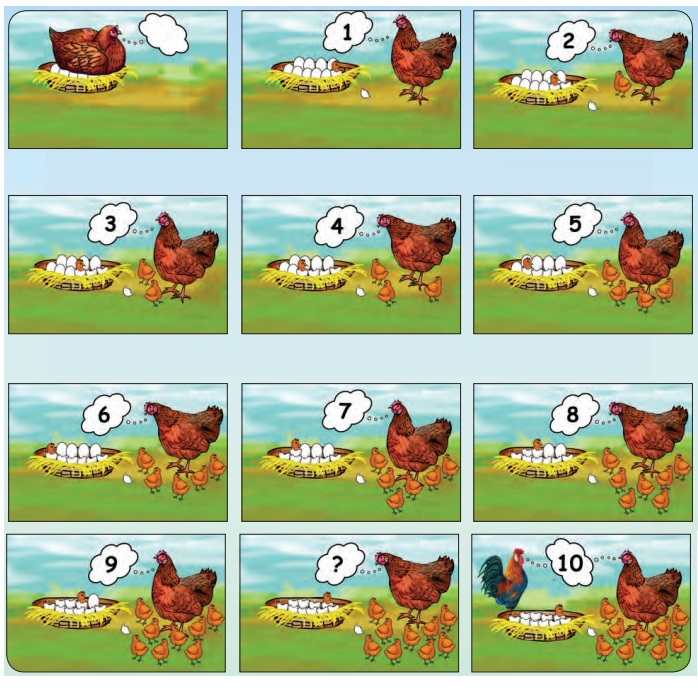Home | | Maths 1st Std | Introduction to number 10

# Introduction to number 10

Teacher's Note: Narrate the above story for the introduction of number 10. Teacher's Note: Try other possible ways of grouping in tens with easily available items such as match sticks, seeds, unused buttons and pebbles etc.,

Introduction to number 10

Travel through

Counting the chicks.Teacher's Note: Narrate the above story for the introduction of number 10.

Learn

Let us learn what comes after 9 by completing the table.10 ones = 1 ten

Learn

Let us make groups of 10.

To make counting of large number of objects easier, we group 10 ones and make one bunch of ten.Practice

Circle groups of 10.Teacher's Note: Try other possible ways of grouping in tens with easily available items such as match sticks, seeds, unused buttons and pebbles etc.,

Practice

Trace and write number 10.Know more

10 in many ways.Think like a Mathematician

Are there any other possible ways of making 10? Say them.

Tags : Numbers | Term 2 Chapter 2 | 1st Maths , 1st Maths : Term 2 Unit 2 : Numbers
Study Material, Lecturing Notes, Assignment, Reference, Wiki description explanation, brief detail
1st Maths : Term 2 Unit 2 : Numbers : Introduction to number 10 | Numbers | Term 2 Chapter 2 | 1st Maths